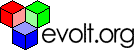Work

# Formatting Numbers For Currency Display And More

Rated 4.06 (Ratings: 3)

#### George

Member info

User since: 21 Apr 2003

Articles written: 2

## Formatting numbers for currency display and more.

Formatting numbers so they conform to a specific format can be

deceivingly tricky. For example, one of the most common tasks is to format a

number for currency display- an integer followed by two decimals. There are

more subtitles in such a task than many might think. In this tutorial, I'll

discuss formatting numbers in JavaScript, such as number rounding,

formatting a number for currency display, and more.

### Number rounding in JavaScript

Lets first talk about number rounding in JavaScript. JavaScript

accomplishes this with the following method:

`Math.round(x)`

Using it, any supplied argument is rounded off to the nearest

integer, and using the ".5" up rule. For example:

`Math.round(25.9) //returns 26Math.round(25.2) //returns 25Math.round(-2.58) //returns -3`

If purging numbers of decimals is all that you require, class

dismissed!

### Taking things a few decimal further

Formatting numbers to specific decimal points still entails

Math.round(), but padded with a little

multiplication and division. See if you can identify the magic formula involved,

with the below examples:

`var original=28.4531) //round "original" to two decimalsvar result=Math.round(original*100)/100  //returns 28.452) // round "original" to 1 decimalvar result=Math.round(original*10)/10  //returns 28.53) //round 8.111111 to 3 decimalsvar result=Math.round(8.111111*1000)/1000  //returns 8.111`

In case you haven't picked up on it, the formula to round any

number to x decimal points is:

1) Multiple the original number by 10^x (10 to the power of x)

2) Apply Math.round() to the result

3) Divide result by 10^x

### Formatting numbers for currency display

Now, lets move on to formatting numbers for currency display in

JavaScript. You may be tempted to simply use number rounding to first shift

the number's decimal places (via multiplication), round it, then shift the

decimal back (via division) to pound the number into your hard earned

dollar, though that won't work in many cases. For example, consider the

number 120. Number rounding certainly won't get you to 120.00.

To easily format numbers for a specific number of trailing decimals or

total digits (aka padding), JavaScript 1.5 introduces the below two nifty

methods:

Methods Description
Number.toFixed(x) Formats any number for "x" number of

trailing decimals. The number is rounded up, and "0"s are used after the

decimal point if needed to create the desired decimal length.
Number.toPrecision(x) Formats any number so it is of "x" length.

Also called significant digits. A decimal point and "0"s are used if

needed to create the desired length.

### Number.toFixed()

The best way to see all the subtleties of

toFixed() is to see it in action:

`var profits=2489.8237profits.toFixed(3) //returns 2489.824 (round up)profits.toFixed(2) //returns 2489.82profits.toFixed(7) //returns 2489.8237000 (padding)`

Displaying any number in currency format can't get any easier!

### Number.toPrecision()

To toPrecision() now:

`var anumber=123.45anumber.toPrecision(6) //returns 123.450 (padding)anumber.toPrecision(4) //returns 123.5 (round up)anumber.toPrecision(2) //returns 1.2e+2 (you figure it out!)`

toPrecision() is useful if your

number must be of a certain length.

### Browser considerations

Now, as noted, the last two methods above are JavaScript 1.5 methods. What this

means is that they'll only work in IE5.5+ and NS6+. The issue of legacy

browsers not performing the desired formatting operation not withstanding,

how do you ensure that these two methods at least degrade well? Well, by

using method detection in your code. For example:

`var profits=2489.8237if (profits.toFixed) //if browser supports toFixed() methodprofits.toFixed(2)`

For those of you who also need to ensure legacy browsers such as IE5 also

perform the desired number formatting operation, well, then it's time to

George is the webmaster of JavaScript Kit (http://www.javascriptkit.com), a comprehensive site featuring tutorials and scripts on JavaScript, DHTML, CSS, and web design. He also mantains the developer's help forum CodingForums.com.

The access keys for this page are: ALT (Control on a Mac) plus:Evolt.org is an all-volunteer resource for web developers made up of a discussion list, a browser archive, and member-submitted articles. This article is the property of its author, please do not redistribute or use elsewhere without checking with the author.